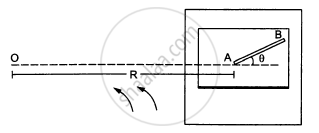Department of Pre-University Education, KarnatakaPUC Karnataka Science Class 11
Advertisement Remove all ads

# A Table with Smooth Horizontal Surface is Fixed in a Cabin that Rotates with a Uniform Angular Velocity ω in a Circular Path of Radius R. - Physics

Sum

A table with smooth horizontal surface is fixed in a cabin that rotates with a uniform angular  velocity ω in a circular path of radius R (In the following figure). A smooth groove AB of length L(<<R) is made the surface of the table. The groove makes an angle θ with the radius OA of the circle in which the cabin rotates. A small particle is kept at the point A in the groove and is released to move at the point A in the groove and is released to move along AB. Find the time taken by the particle to reach the point B.Advertisement Remove all ads

#### Solution

Let the mass of the particle be m.
Radius of the path = R
Angular velocity = ω
Force experienced by the particle = mω2R
The component of force mRω2 along the line AB (making an angle with the radius) provides the necessary force to the particle to move along AB.

$\therefore m \omega^2 R \cos\theta = ma$

$\Rightarrow a = \omega^2 R\cos\theta$

Let the time taken by the particle to reach the point B be t.

$\text { On using equation of motion, we get : }$

$L = ut + \frac{1}{2}a t^2$

$\Rightarrow L = \frac{1}{2} \omega^2 R\cos\theta t^2$

$\Rightarrow t^2 = \frac{2L}{\omega^2 R\cos\theta}$

$\Rightarrow t = \sqrt{\frac{2L}{\omega^2 R\cos\theta}}$

Is there an error in this question or solution?
Advertisement Remove all ads

#### APPEARS IN

HC Verma Class 11, 12 Concepts of Physics 1
Chapter 7 Circular Motion
Q 28 | Page 116
Advertisement Remove all ads
Advertisement Remove all ads
Share
Notifications

View all notifications

Forgot password?